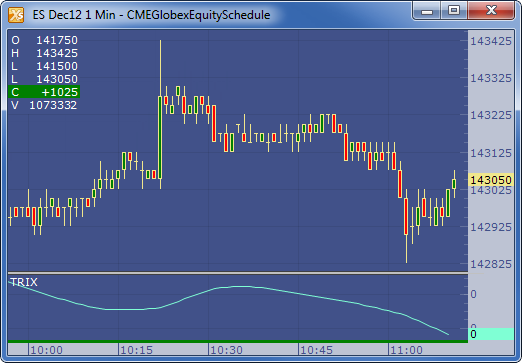← Back to X_TRADER® Help Library

### X_STUDY® Documentation

#### Triple Exponential Moving Average Oscillator (TRIX)

##### Description

The Triple Exponential Moving Average Oscillator (TRIX) by Jack Hutson is a momentum indicator that oscillates around zero. It displays the percentage rate of change between two triple smoothed exponential moving averages.

##### Formula

EMA1 = EMA1n-1 + ((2 / (n + 1)) * (Pn - EMA1n-1))

EMA2 = EMA2n-1 + ((2 / (n + 1)) * (EMA1n - EMA2n-1))

EMA3 = EMA3n-1 + ((2 / (n + 1)) * (EMA2n - EMA3n-1))

TRIX = (EMA3n - EMA3n-1 ) / EMA3n-1

Where:

• Pn =the current price.
• EMA1n-1 = the exponential moving average value of n periods back
• EMA2n-1 = the exponential moving average value of n periods back
• EMA3n-1 = the exponential moving average value of n periods back
##### Example